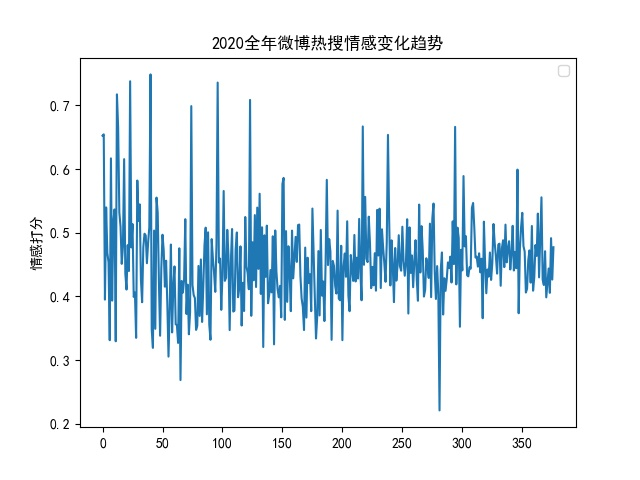# 热搜抓取

["getclosesttime",["2021-01-20T23:08:02"]]



# 获取指定日期对应的timeId
cacheKey = date.strftime('%Y-%m-%d')
records = list(store.find(TABLE_TIME_ID, {'date': cacheKey}))
if len(records) > 0:
return records['timeId']
else:
data = "[\"getclosesttime\",[\"{d}\"]]".format(d=cacheKey)
data = base64.b64encode(data.encode('utf-8'))
url = 'https://www.weibotop.cn/apis/androidrouter/?versioncode=1&=&data=' + str(data, 'utf-8')
store.insert(TABLE_TIME_ID, [{'date': cacheKey, 'timeId': timeId }])
return timeId

# 获取指定timeId对应的热搜
records = list(store.find(TABLE_TRENDING, {'timeId': timeId}))
if len(records) > 0:
return records
else:
url = 'https://www.eecso.com/test/weibo/apis/currentitems.php?timeid=' + timeId
trendings = list(map(lambda x:{'title':x, 'createdDate':x, 'updatedDate':x, 'rank':int(x)}, data))
for trending in trendings:
trending['timeId'] = timeId
trending['href'] = 'https://s.weibo.com/weibo?q=' + trending['title']
trending['createdDate'] = datetime.datetime.strptime(trending['createdDate'], '%Y-%m-%d %H:%M:%S')
trending['updatedDate'] = datetime.datetime.strptime(trending['updatedDate'], '%Y-%m-%d %H:%M:%S')
store.insert(TABLE_TRENDING, trendings)
return trendings